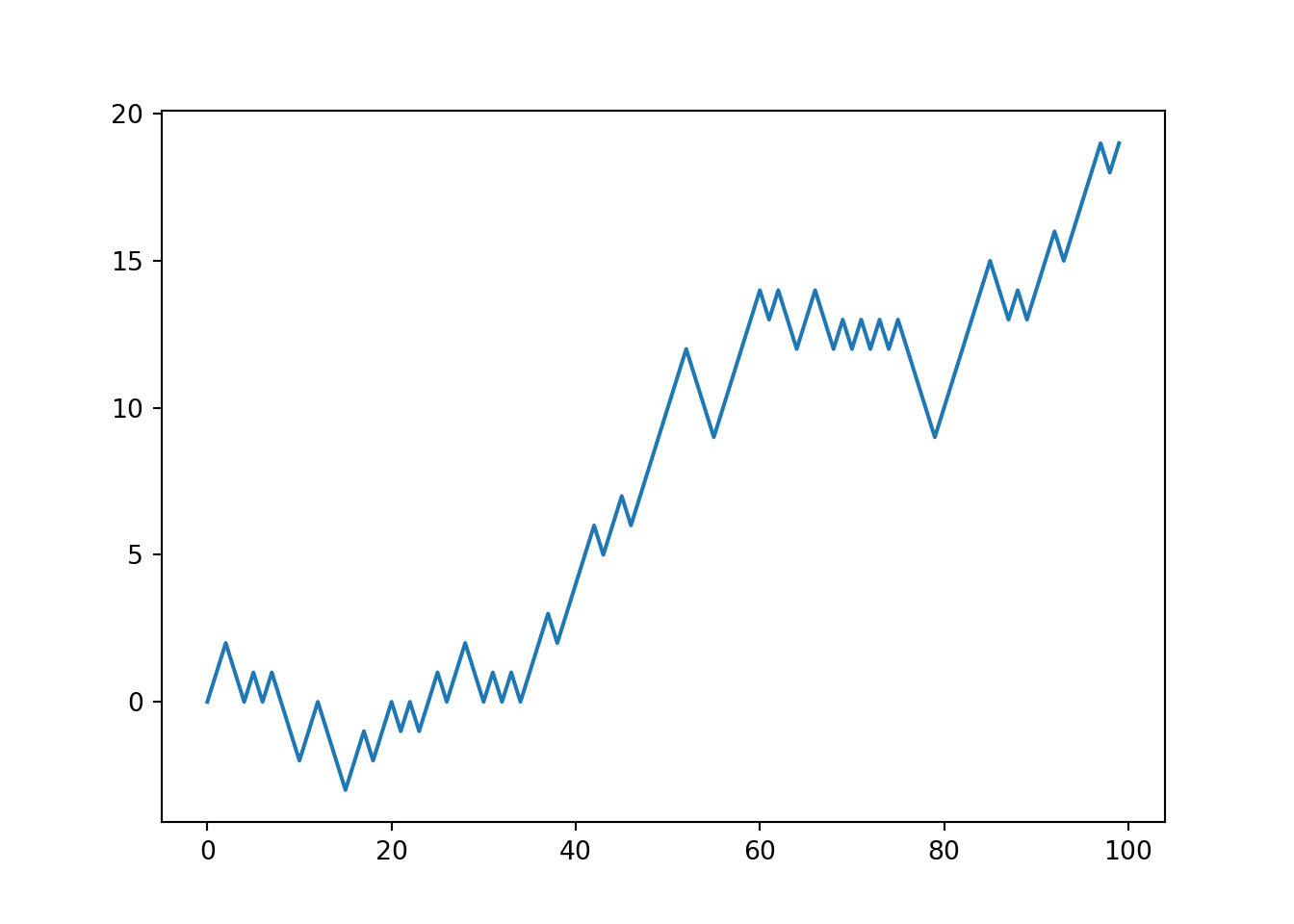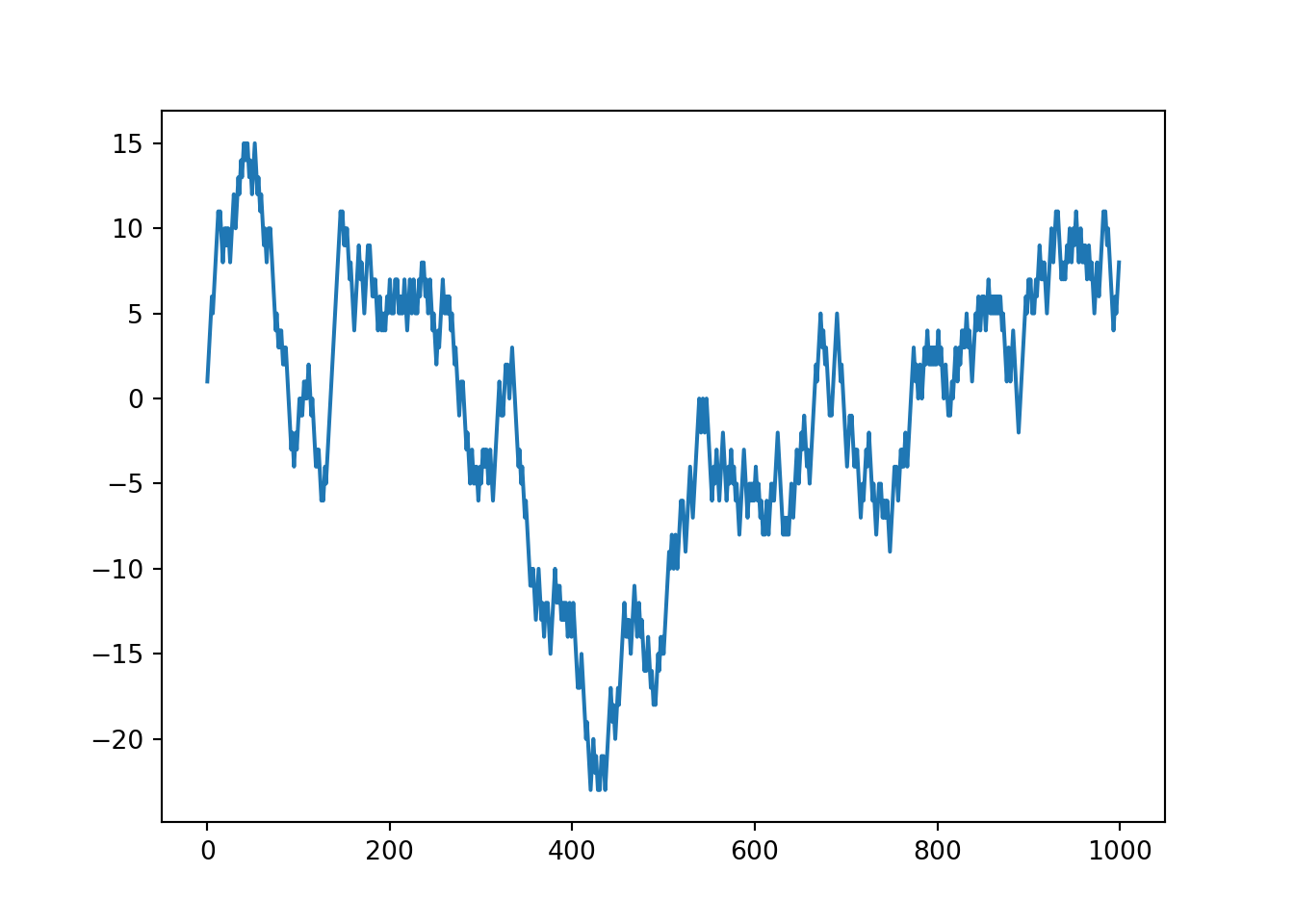## 1. What are Random Walks?

• In short, a random walk is a stochastic process.
• In this example, we will consider a simple random walk starting at 0 with steps of 1 and -1 occuring with equal probability.
import random

position = 0
walk = [position]
steps = 1000

for i in range(steps):
step = 1 if random.randint(0, 1) else -1
position += step
walk.append(position)
• Now let’s plot our walk!
import matplotlib.pyplot as plt

plt.plot(walk[:100])## 2. Using NumPy for Simulating Random Walks

• walk is simply the cumulative sum of the random steps and could be evaluated as an array expression.
import numpy as np
np.random.seed(430)

nsteps = 1000
draws = np.random.randint(0, 2, size=nsteps)
steps = np.where(draws > 0, 1, -1)
walk = steps.cumsum()
• Note: we have to use np.random.randint() to generate an array of random integers. random.randint() only returns 1 number at the time.

• Since we use 2 different random functions, even if we set the same seed, we won’t get the same result (because the mechanisms inside the functions work differently).

• Here is the plot of new generated walk:

plt.plot(walk)• From this we can begin to extract statistics like the minimum and maximum value along the walk’s trajectory:
walk.min()
## -23
walk.max()
## 15
• First crossing time = the step at which the random walk reaches a particular value. This is a more advanced statistic.
• Let’s say we want to know how long it takes the random walk to get at least 10 steps away from the starting point in either direction.
(np.abs(walk) >= 10).argmax()
## 11
• np.abs(walk) >= 10 gives us a boolean array indicating where the walk has reached or exceeded 10.
• argmax() returns the first index of the maximum value of the array. In this case, it returns the index of the first True value.

## 3. Simulating Many Random Walks at Once

• Say our goal is to generate 5000 random walks at once! How do we do that?
nwalks = 5000
nsteps = 1000
draws = np.random.randint(0, 2, size=(nwalks, nsteps))
steps = np.where(draws > 0, 1, -1)
walks = steps.cumsum(1) # sum across the columns
walks
## array([[ -1,   0,   1, ..., -28, -27, -26],
##        [ -1,   0,  -1, ...,  54,  55,  56],
##        [ -1,  -2,  -3, ...,   2,   3,   2],
##        ...,
##        [ -1,   0,  -1, ..., -22, -21, -22],
##        [  1,   2,   1, ...,  48,  49,  48],
##        [  1,   0,   1, ..., -38, -39, -38]])
• Out of these walks, let’s compute the minimum crossing time to 30 or -30!
• Now, note that not all of 5000 walks reach 30.
hits30 = (np.abs(walks) >= 30).any(1)
hits30
## array([ True,  True, False, ...,  True,  True,  True])
• any(1) returns True if at least 1 of the values of the row is True.
# number of walks that hit 30 or -30
hits30.sum()
## 3336
# estimate for probability a walk hitting 30 in either direction
hits30.sum()/nwalks
## 0.6672
• Use the boolean array hist30 to select only the walks that actually hit 30 or -30! Then use argmax() across axis 1 (the column) to get the crossing times:
crossing_times = (np.abs(walks[hits30]) >= 30).argmax(1)
crossing_times.mean()
## 511.1636690647482

This lecture note is modified from Chapter 4 of Wes McKinney’s Python for Data Analysis 2nd Ed.